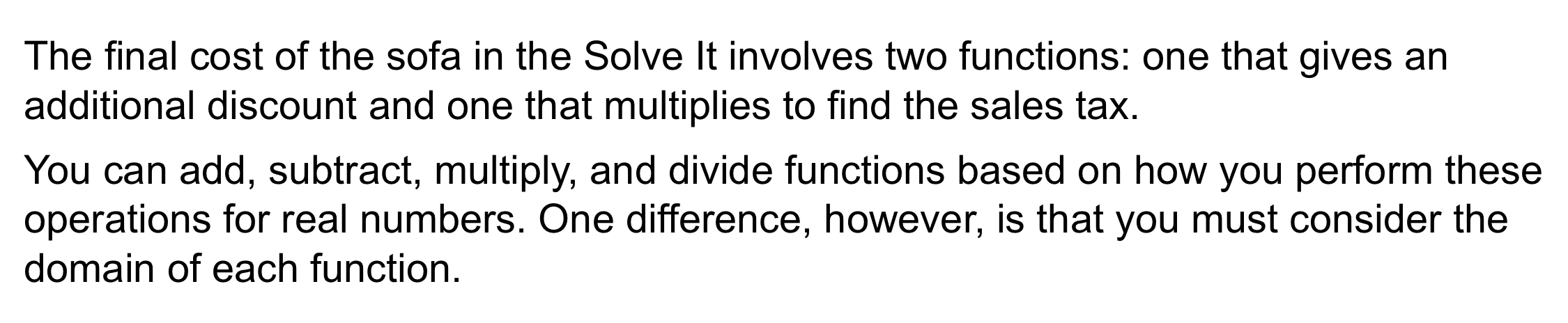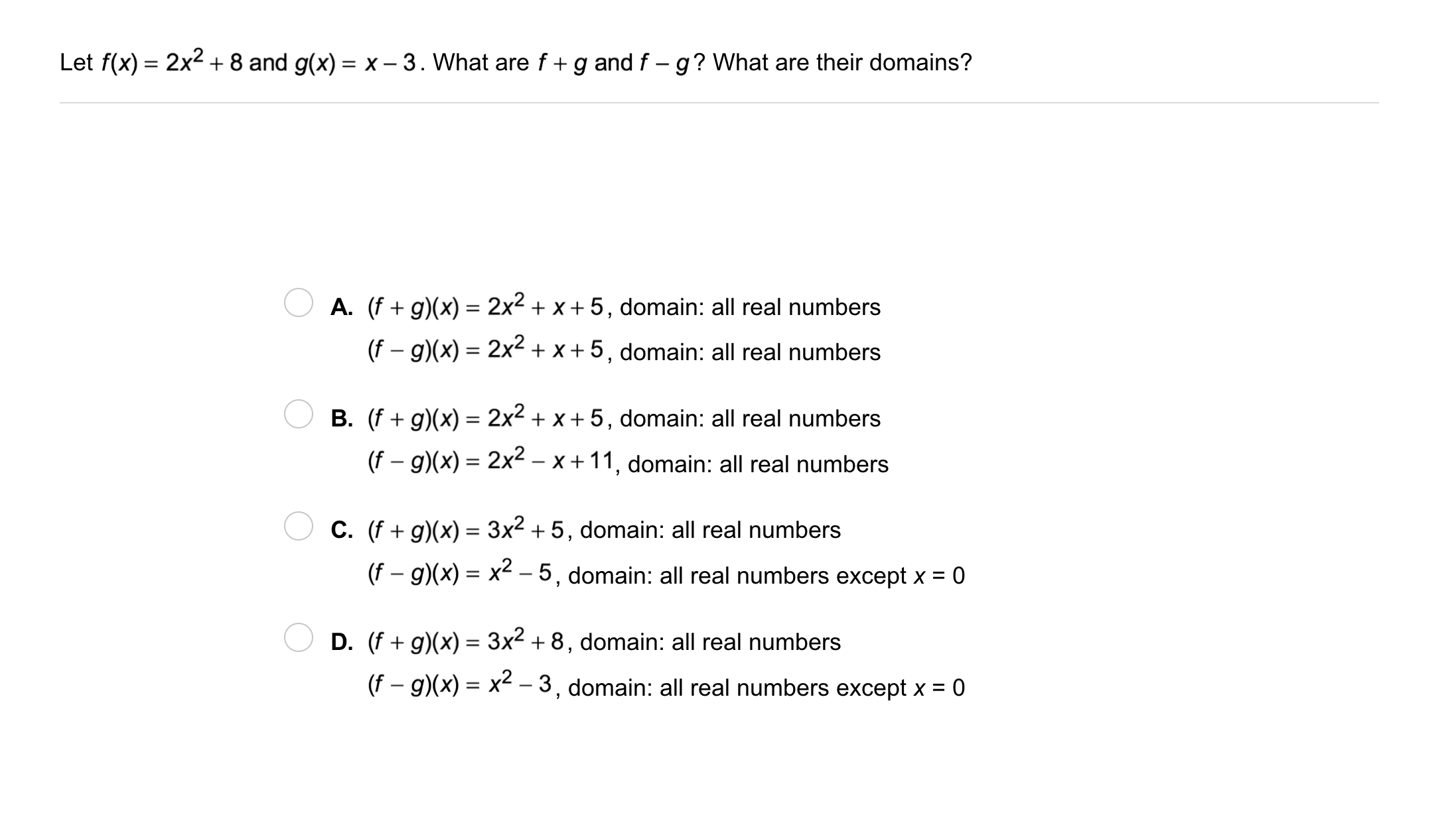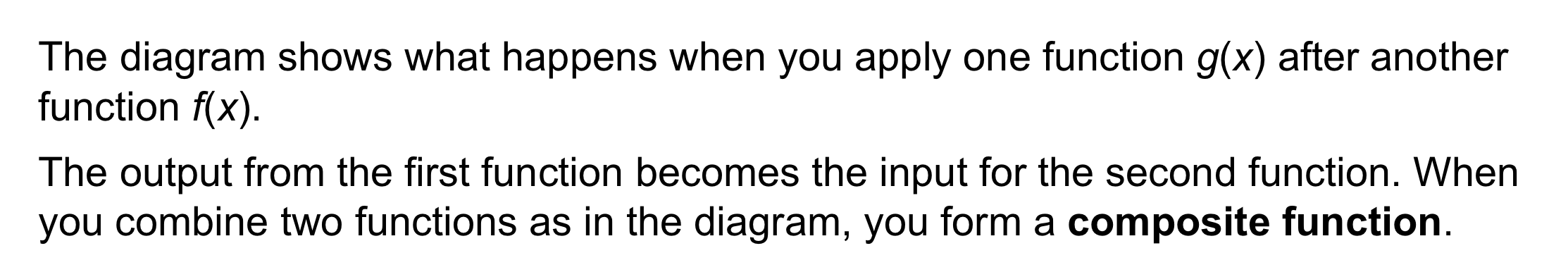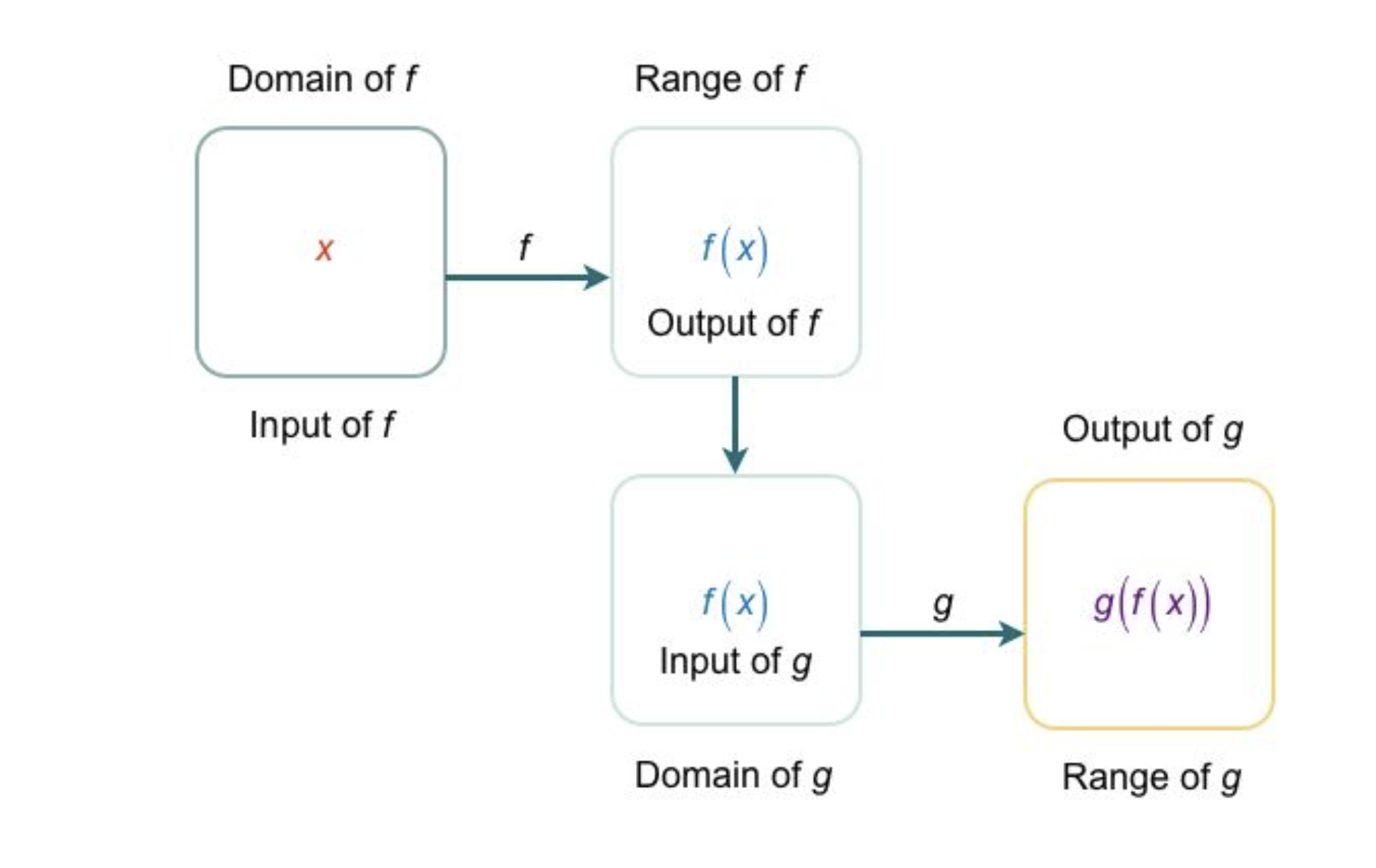Algebra 2 6-6 Guided Practice: Function Operations
starstarstarstarstarstarstarstarstarstar
by Matthew Richardson
| 11 Questions1
10
Solve It! You want to buy a sofa that was marked down by \$100 before the clearance discount was applied.

The furniture store may add the 5% sales tax before applying the additional discount, or it may add the sales tax after applying the additional discount. Which way is better for you, the customer?
After
Before
2
10
Solve It! In the above item, how much do you save by choosing the less expensive option?
Enter your response in this format: \$12.30a
Take Note: Take a moment to record the function operations in your notes.3
3
10
Problem 1 Got It?
A
B
C
D4
4
10
Problem 2 Got It?
A
B
C5
5
10
Take Note: What is a composite function ?6
6
10
Problem 3 Got It?
A
B
C
D
7
10
Problem 4 Got It? A store is offering a 15% discount on all items. Also, employees get a 20% discount (20% off original price(s)). Write a composite function to model taking the 15% discount and then the 20% discount.
Let D(x) = cost after applying the 15% discount, E(x) = cost after applying the 20% employee discount, and x = cost of item(s). Then D(x) = 0.85x and E(x) = 0.80x. (ED)(x) = 0.68x.
Let D(x) = cost after applying the 15% discount, E(x) = cost after applying the 20% employee discount, and x = cost of item(s). Then D(x) = 0.80x and E(x) = 0.85x. (ED)(x) = 0.68x.
8
10
Problem 4 Got It? A store is offering a 15% discount on all items. Also, employees get a 20% discount (20% off original price(s)). Write a composite function to model taking the 20% discount and then the 15% discount.
Let D(x) = cost after applying the 15% discount, E(x) = cost after applying the 20% employee discount, and x = cost of item(s). Then D(x) = 0.85x and E(x) = 0.80x. (DE)(x) = 0.68x.
Let D(x) = cost after applying the 15% discount, E(x) = cost after applying the 20% employee discount, and x = cost of item(s). Then D(x) = 0.80x and E(x) = 0.85x. (DE)(x) = 0.68x.
9
10
Problem 4 Got It? Reasoning: In the scenario above, which order of discounts results in a lower cost to the employee?
The total discounts are the same, so it does not matter which discount you take first.
Taking the 20% discount first results in a lower price.
Taking the 15% discount first results in a lower price.11
11
10
Take Note: Summarize the content of this lesson.